# Conditional styles

Tags

You can use PHP to control the way a page looks, by changing the styles it uses.

### How many dogs?

Try this link to a PHP page that evaluates the number of dogs you have. Number of dogs is passed in as a URL parameter names `dogs`. Here, there are no dogs.

http://webappexamples.skilling.us/html/conditional-styles/how-many-dogs.php?dogs=0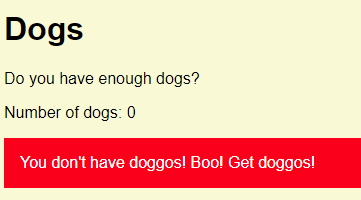Try a link for one dog:

http://webappexamples.skilling.us/html/conditional-styles/how-many-dogs.php?dogs=1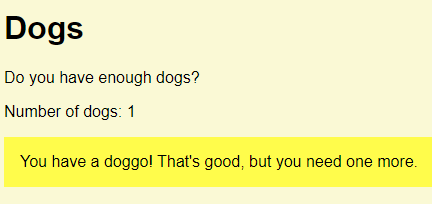Now for two dogs:

http://webappexamples.skilling.us/html/conditional-styles/how-many-dogs.php?dogs=2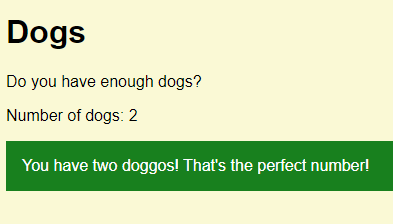Finally, three dogs.

http://webappexamples.skilling.us/html/conditional-styles/how-many-dogs.php?dogs=3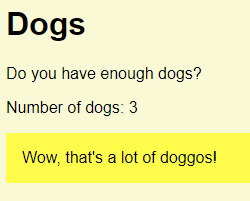### What's the HTML?

Check the HTML the program makes when there are no dogs:

• ...
• <link rel="stylesheet" href="styles.css">
• ...
• <p>Number of dogs: 0</p>
• <p class='argh'>You don't have doggos! Boo! Get doggos!</p>
• ...

Now when there are two dogs:

• ...
• <link rel="stylesheet" href="styles.css">
• ...
• <p>Number of dogs: 2</p>
• <p class='good'>You have two doggos! That's the perfect number!</p>
• ...

The class is `argh` in one case, and `good` in another.

### `if`

Here's the code:

1. <?php
2. \$dogs = \$_GET['dogs'];
3. // Compute message, and CSS class.
4. \$message = '';
5. \$class = '';
6. if (\$dogs < 1) {
7.     \$message = "You don't have doggos! Boo! Get doggos!";
8.     \$class = 'argh';
9. }
10. else if (\$dogs == 1) {
11.     \$message = "You have a doggo! That's good, but you need one more.";
12.     \$class = 'fair';
13. }
14. else if (\$dogs == 2) {
15.     \$message = "You have two doggos! That's the perfect number!";
16.     \$class = 'good';
17. }
18. else {
19.     \$message = "Wow, that's a lot of doggos!";
20.     \$class = 'fair';
21. }
22. ?><!DOCTYPE html>
23. <html lang="en">
25.         <meta charset="UTF-8">
26.         <title>Dogs</title>
27.         <meta name="viewport" content="width=device-width, initial-scale=1, shrink-to-fit=no">
28.         <link rel="stylesheet" href="styles.css">
30.     <body>
31.         <h1>Dogs</h1>
32.         <p>Do you have enough dogs?</p>
33.         <p>Number of dogs: <?= \$dogs ?></p>
34.         <p class="<?= \$class ?>"><?= \$message ?></p>
35.     </body>
36. </html>

Line 2 gets the number of dogs from the URL:

• \$dogs = \$_GET['dogs'];

line 3 is:

• // Compute message, and CSS class.

That's a comment. Comments are Good Things.

Let's skip down a bit, and look at the output. Line 33 outputs the number of dogs:

• print "<p>Number of dogs: \$dogs</p>\n";

The `\n` thing on the end adds a new line in the HTML, like hitting the Enter key. It doesn't affect what the user sees, but it does affect the HTML. Here's the HTML without the `\n`...

• <p>Number of dogs: 0</p><p class='argh'>You don't have doggos! Boo! Get doggos!</p>

... and with it...

• <p>Number of dogs: 0</p>
• <p class='argh'>You don't have doggos! Boo! Get doggos!</p>

As you can see, adding the new-line thing makes the HTML easier to understand. That's particularly useful when debugging.

`\n` only works with you use double quotes (").

Works: `print "<p>You rock!</p>\n";`

Doesn't work: `print '<p>You rock!</p>\n';`

Line 34 is the main output:

• <p class="<?= \$class ?>"><?= \$message ?></p>

That `<?= \$variable ?>` is shorthand for `<?php print \$variable; ?>`.

Notice how simple that code is. It outputs two variables: `\$class`, and `\$message`.

The statement prints some HTML. Here's what it prints, for one doggo.

• <p class="fair">You have a doggo! That's good, but you need one more.</p>

`\$class` is "fair", and `\$message` is "You have a doggo! That's good, but you need one more."

Here's what it prints, for two doggos:

• <p class="good">You have two doggos! That's the perfect number!</p>

In that case, `\$class` is "good", and `\$message` is "You have two doggos! That's the perfect number!"

The program works by setting `\$class` and `\$message` to the right values. Then, when it gets to the output section, the code to use those variables is simple:

• <p class="<?= \$class ?>"><?= \$message ?></p>

Most of the program's logic is working out the two variables. We put that code at the top of the PHP file. That's common. PHP programs usually look like this: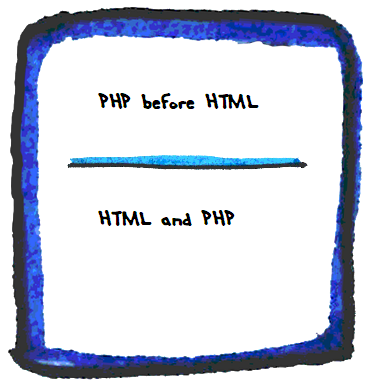We do most of the work at the top, so the output can be simple. The top bit fills some variables, that are just output at the bottom.

Pattern

Put code for complex calculations in the top part of your program. That code puts values in variables. The bottom bit makes HTML using those variables.

Here's the code that sets the variables:

1. // Compute message, and CSS class.
2. \$message = '';
3. \$class = '';
4. if (\$dogs < 1) {
5.     \$message = "You don't have doggos! Boo! Get doggos!";
6.     \$class = 'argh';
7. }
8. else if (\$dogs == 1) {
9.     \$message = "You have a doggo! That's good, but you need one more.";
10.     \$class = 'fair';
11. }
12. else if (\$dogs == 2) {
13.     \$message = "You have two doggos! That's the perfect number!";
14.     \$class = 'good';
15. }
16. else {
17.     \$message = "Wow, that's a lot of doggos!";
18.     \$class = 'fair';
19. }
20. // Output.
21. print "<p class='\$class'>\$message</p>\n";

First, we make the variables, and set them to empty strings.

• \$message = '';
• \$class = '';

You don't have to do this; the program would work just fine without these lines. I added the lines as documentation, a reminder to me that the program works by setting the values of these variables. It also gives me a place to hang comments, if I wanted.

Here's the first `if`:

• if (\$dogs < 1) {
•     \$message = "You don't have doggos! Boo! Get doggos!";
•     \$class = 'argh';
• }

There's a test in parentheses (the () things). It's either true, or false. (There's a bit more to it. Later.) The parens are not optional. They must be there.

If the test is true, the code between the braces ({}) runs. If the test is false, the code is skipped. So the close brace (}) acts like `EndIf` in VBA.

Reflect

This line uses double quotes ("):

• \$message = "You don't have doggos! Boo! Get doggos!";

Why? There's no \n at the end.

If you were logged in as a student, the lesson would pause here, and you'd be asked to type in a response. If you want to try that out, ask for an account on this site.Ethan

The message has a single quote (') in it, in the word "don't". If you used single quotes around the string...

• \$message = 'You don't have doggos! Boo! Get doggos!';

...PHP might think the ' in "don't" was the end of the string.

Right!

The next code is:

• else if (\$dogs == 1) {
•     \$message = "You have a doggo! That's good, but you need one more.";
•     \$class = 'fair';
• }Ethan

There's an extra = in the `if`, right? It should be =, not ==.

No! It's right as it is. In VBA, we might write the code like this:

• ElseIf sDogs = 1 Then
•   tMessage = "You have a doggo! That's good, but you need one more."
•   tClass = "fair";
• EndIf

So = has two meanings in VBA. In an `If`, = means "compare." In the lines below the `If`, = means "assign," that is, take the thing on the right, and put it into the thing on the left.

PHP isn't like that. "Compare to" and "assign" are written differently. Use == for "compare to." That will be true or false. Use = for assign.So, what would happen if you typed `if (\$dogs = 1) {`, with just one =?

PHP would put 1 into `\$dogs`, overwriting what was there! You'd get the wrong output, and lose the value of `\$dog` as well.

Not good.

Warning

Use `==` in `if` tests, not `=`.

When there's only one case left, we can leave off the `if`.

• else {
•     \$message = "Wow, that's a lot of doggos!";
•     \$class = 'fair';
• }

Overall, the pattern is something like:

• \$variable = initial value;
• if (test) {
•   \$variable = something;
• }
• else if (another test) {
•   \$variable = something else;
• }
• ...
• else {
•   \$variable = something else again;
• }
• Do something with \$variable;

Another example:

• \$message = '';
• if (\$age >= 21) {
•   \$message = 'Welcome to the club!';
• }
• else {
•   \$message = 'Sorry, you must be at least 21 to enter the club.';
• }
• print "<p>\$message</p>\n";

The same thing:

• \$message = 'Welcome to the club!';
• if (\$age < 21) {
•   \$message = 'Sorry, you must be at least 21 to enter the club.';
• }
• print "<p>\$message</p>\n";

In the second one, the variable's initial value is set to one of the options. That value is replaced in the `if`, if the condition is met.

Another example:

• \$salesTaxRate = 0;
• // Make \$state lowercase, and trim spaces.
• \$state = strtolower(trim(\$state));
• if (\$state == 'mi') {
•   \$salesTaxRate = 0.06;
• }
• else if (\$state == 'in') {
•   \$salesTaxRate = 0.07;
• }
• else if (\$state == 'oh') {
•   \$salesTaxRate = 0.0575;
• }
• else if (\$state == 'il') {
•   \$salesTaxRate = 0.0625;
• }
• else {
•     print "<p>Unknown state: \$state</p>\n";
•     exit();
• }
• \$salesTax = \$total * \$salesTaxRate;

In the second line, the variable `\$state` is trimmed of spaces, and converted to lowercase, before it is tested. We did the same thing in VBA.

If `\$state` is invalid, the code shows an error message in the HTML, and stops. `exit()` is the same as `End` in VBA. We'll improve validation later.

### It's a pattern

This...

• \$variable = initial value;
• if (test) {
•   \$variable = something;
• }
• else if (another test) {
•   \$variable = something else;
• }
• ...
• else {
•   \$variable = something else again;
• }
• Do something with \$variable;

... is common in programming, in any language. You can use it again and again, in different ways. Let's call it the multiway if pattern.

Pattern

Make a chain of `if`s and `elseif`s for a variable that can have several different values.

It goes into the pattern catalog. The catalog is a list of patterns you can reuse in your own code. If you're writing a program, and aren't sure what to do, check out the catalog. There's a link to it under Lists in the main menu.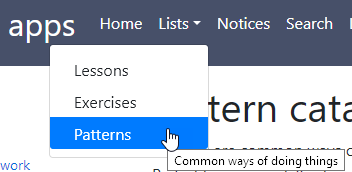### Indenting and braces

We use some coding standards in the course.

Code in `if`s and loops must be indented, by 2 or 4 spaces. Either is fine. You must be consistent within each file, though. Like this:

• if (\$hasExploded) {
•     print "Boom!";
• }
• else {
•     print "Nothing";
• }

Also, don't leave out the braces. This would work...

• if (\$hasExploded)
•     print "Boom!";
• else
•     print "Nothing";

... but don't do it! You're asking for bugs. Always put the braces in.

Check the coding standards page for all the deets.

### Test for missing GET parameters

Here's some code from before:

• \$dogs = \$_GET['dogs'];

What if you don't give `dogs`, or misspell it?

• something.php?logs=2

You'll get an error: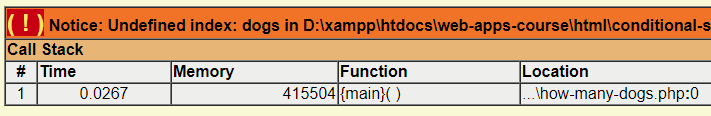If a non-geek sees that, their head might explode.

Let's make a more friendly error message, like this: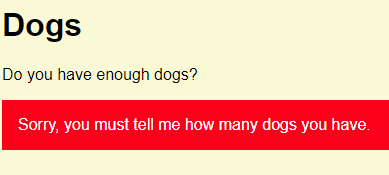It's still an error, but the message won't freak out the norms.

Here's some code. There are a few things going on, so pay attention to it. I'm just showing the new stuff.

1. <?php
2. \$errorMessage = '';
3. if (!isset(\$_GET['dogs'])) {
4.     \$errorMessage = 'Sorry, you must tell me how many dogs you have.';
5. }
6. if (\$errorMessage == '') {
7.     \$dogs = \$_GET['dogs'];
8. }
9. ?><!DOCTYPE html>
10. <html lang="en">
11.   ...
12.   <p>Do you have enough dogs?</p>
13.   <?php
14.   if (\$errorMessage != '') {
15.       print "<p class='argh'>\$errorMessage</p>\n";
16.   }
17.   else {
18.       // Show number of dogs.
19.       print "<p>Number of dogs: \$dogs</p>\n";
20.       ...
21.   }
22.   ?>
23. </body>
24. </html>

Line 2 makes a variable called `\$errorMessage`, and makes it MT. We'll use `\$errorMessage` for two purposes:

• Hold error messages.
• Show whether there were any errors.

We'll use `\$errorMessage` as a flag. If it's MT, there have been no errors. If it isn't MT, there have been errors.

Here a use example:

• if (\$errorMessage == '') {
•   print 'No errors';
• }
• else {
•   print "Something went wrong: \$errorMessage";
• }

OK, back to the code:

1. <?php
2. \$errorMessage = '';
3. if (!isset(\$_GET['dogs'])) {
4.     \$errorMessage = 'Sorry, you must tell me how many dogs you have.';
5. }
6. if (\$errorMessage == '') {
7.     \$dogs = \$_GET['dogs'];
8. }
9. ?><!DOCTYPE html>
10. <html lang="en">
11.   ...
12.   <p>Do you have enough dogs?</p>
13.   <?php
14.   if (\$errorMessage != '') {
15.       print "<p class='argh'>\$errorMessage</p>\n";
16.   }
17.   else {
18.       // Show number of dogs.
19.       print "<p>Number of dogs: \$dogs</p>\n";
20.       ...
21.   }
22.   ?>
23. </body>
24. </html>

Line 3 uses the `isset()` function. It's true of the parameter exists, false if it does not. If there's nothing in GET with the index `dogs`, we set `\$errorMessage` to..., well, an error message.

Line 6 tests whether there are no errors. I could have used an `else`:

• if (!isset(\$_GET['dogs'])) {
•     \$errorMessage = 'Sorry, you must tell me how many dogs you have.';
• }
• else {
•     \$dogs = \$_GET['dogs'];
• }

• if (!isset(\$_GET['dogs'])) {
•     \$errorMessage = 'Sorry, you must tell me how many dogs you have.';
• }
• if (\$errorMessage == '') {
•     \$dogs = \$_GET['dogs'];
• }

I used second `if`, since you'll be seeing that a lot. It's a quick way of testing whether any errors have happened.

Here's the code again:

1. <?php
2. \$errorMessage = '';
3. if (!isset(\$_GET['dogs'])) {
4.     \$errorMessage = 'Sorry, you must tell me how many dogs you have.';
5. }
6. if (\$errorMessage == '') {
7.     \$dogs = \$_GET['dogs'];
8. }
9. ?><!DOCTYPE html>
10. <html lang="en">
11.   ...
12.   <p>Do you have enough dogs?</p>
13.   <?php
14.   if (\$errorMessage != '') {
15.       print "<p class='argh'>\$errorMessage</p>\n";
16.   }
17.   else {
18.       // Show number of dogs.
19.       print "<p>Number of dogs: \$dogs</p>\n";
20.       ...
21.   }
22.   ?>
23. </body>
24. </html>

Line 14 is down in the HTML output area. If there's an error message, show it. If not, do the rest of the output.

We'll use `\$errorMessage` this way throughout the course. It's handy pattern.

Pattern

Use a variable containing error messages as a flag. If it's MT, no errors.

### Up next

Let's switch gears a bit, and see how PHPStorm helps use write and debug programs.